# Python – Create UIs for prototyping Machine Learning model with Gradio

Gradio is an open-source python library which allows you to quickly create easy to use, customizable UI components for your ML model, any API, or any arbitrary function in just a few lines of code. You can integrate the GUI directly into your Python notebook, or you can share the link to anyone.

Requirements :

``\$ pip install gradio``

Example :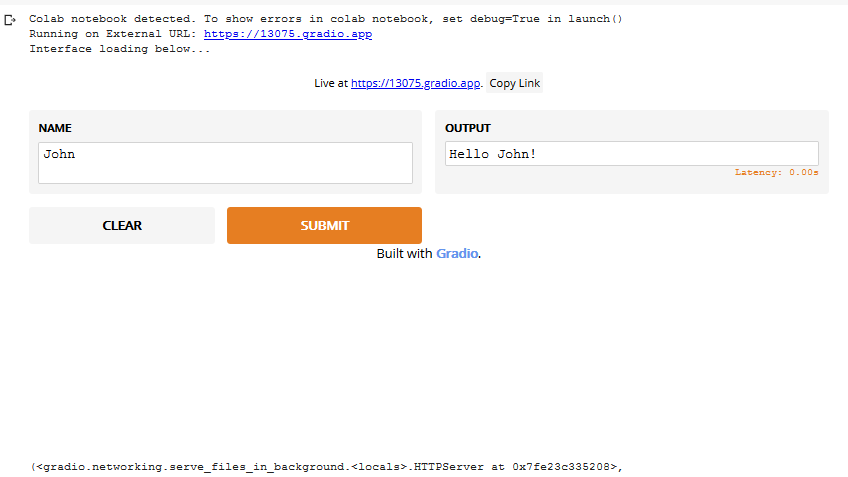We can create interfaces with Gradio using `gradio.Interface() `function.
```gradio.Interface(self, fn, inputs, outputs, examples=None, live=False,          capture_session=False, title=None, description=None)```

Parameters :

• fn: (Callable)the function to wrap an interface.
• inputs: (Union[str, List[Union[str, AbstractInput]]]) a single Gradio input component, or list of Gradio input components.
• outputs: (Union[str, List[Union[str, AbstractOutput]]]) a single Gradio output component, or list of Gradio output components.
• live: (bool) whether the interface should automatically reload on change.
• capture_session: (bool) if True, captures the default graph and session (needed for Tensorflow 1.x)
• title: (str) a title for the interface; if provided, appears above the input and output components.
• description: (str) a description for the interface; if provided, appears above the input and output components.
• examples: (List[List[Any]]) sample inputs for the function; if provided, appears below the UI components and can be used to populate the interface. Should be nested list, in which the outer list consists of samples and each inner list consists of an input corresponding to each input component.

UI for the interface can be generated by `gradio.Interface, launch()` function.
`gradio.Interface.launch(self, share=False)`

Parameters :

`share: (bool) - whether to create a publicly shareable link from your computer for the interface.`

There are several Input and Output Component given for the inputs and outputs parameter of `gradio.Interface()`.

Code: function which returns the factorial of a number.

 `def` `factorial(integer): ` `    ``""" Returns factorial of the given integer"""`  `    ``n ``=` `int``(integer) ` `    ``if` `n<``=``1``: ` `        ``return` `1` `    ``fact``=``1` `    ``for` `i ``in` `range``(``1``, n``+``1``): ` `        ``fact``*``=``i ` `    ``return` `fact `

Now, to wrap this function with gradio interface write following code in the same file.

 `import` `gradio ` `gradio.Interface(factorial, inputs``=``"text"``, outputs``=``"text"``).launch(share``=``True``) `

When you run the above code cells in a jupyter notebook. It will generate a UI like this: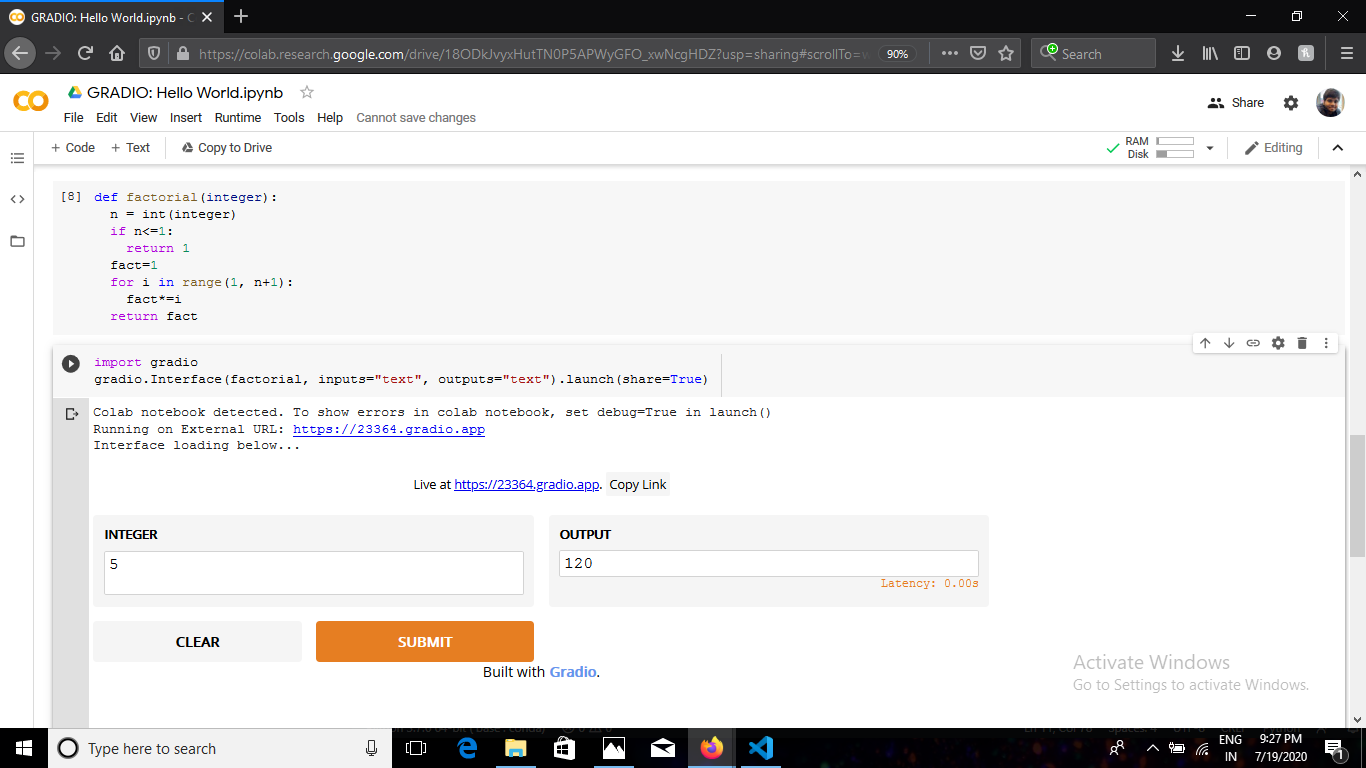You can also copy the link and share that to anyone, it will open the same UI in the browser. Now, we’ll show you how to make an interface for a Machine Learning model.
For the demo, we’ll load a pre-trained Inception Net Image Classification model with tensorflow. Since this is an Image Classification model we’ll use Image input interface. We’ll output a dictionary of labels and their corresponding confidence scores with the Label output interface.

Code:

 `# imported necessary libraries ` `import` `gradio as gr ` `import` `tensorflow as tf ` `import` `numpy as np ` `import` `requests ` ` `  `# loading the model ` `inception_net ``=` `tf.keras.applications.InceptionV3()  ` ` `  `# Download human-readable labels. ` `response ``=` `requests.get(``"https://git.io/JJkYN"``) ` `labels ``=` `response.text.split(``"\n"``) ` ` `  `def` `classify_image(image): ` `    ``""" Returns a dictionary with key as label and values  ` `    ``as the predicted confidence for that label"""` `    ``# reshaping the image ` `    ``image ``=` `image.reshape((``-``1``, ``299``, ``299``, ``3``))  ` `    ``# preprocessing the image for inception_v3 ` `    ``image ``=` `tf.keras.applications.inception_v3.preprocess_input(image)  ` `    ``# predicting the output ` `    ``prediction ``=` `inception_net.predict(image).flatten() ` `    ``return` `{labels[i]: ``float``(prediction[i]) ``for` `i ``in` `range``(``1000``)} ` ` `  `# initializing the input component ` `image ``=` `gr.inputs.Image(shape ``=` `(``299``, ``299``, ``3``))  ` `# initializing the output component  ` `label ``=` `gr.outputs.Label(num_top_classes ``=` `3``)  ` ` `  `# launching the interface ` `gr.Interface(fn ``=` `classify_image, inputs ``=` `image,  ` `             ``outputs ``=` `label, capture_session ``=` `True``).launch() `

When you run the above code cell it will generate the UI like this: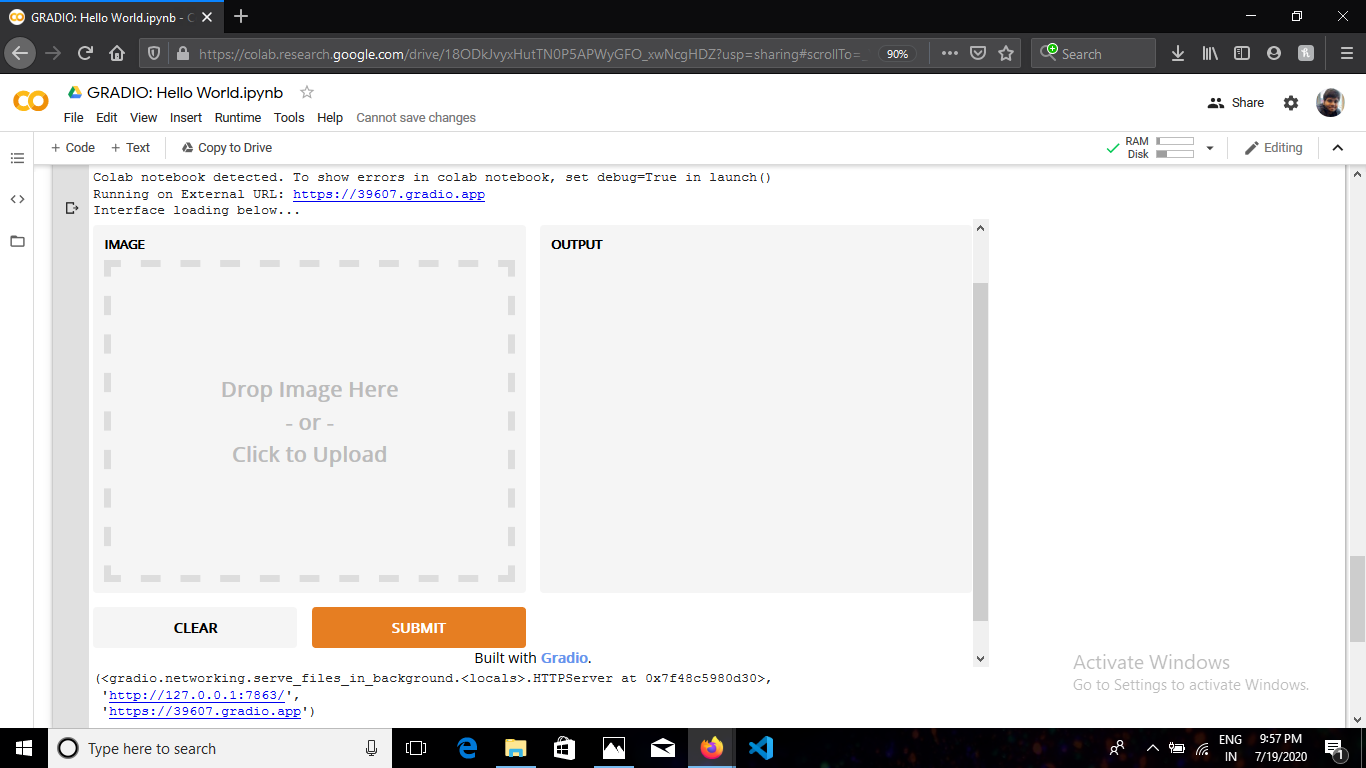Here you can drag and drop the image in the left section of UI and click submit you will get the result like: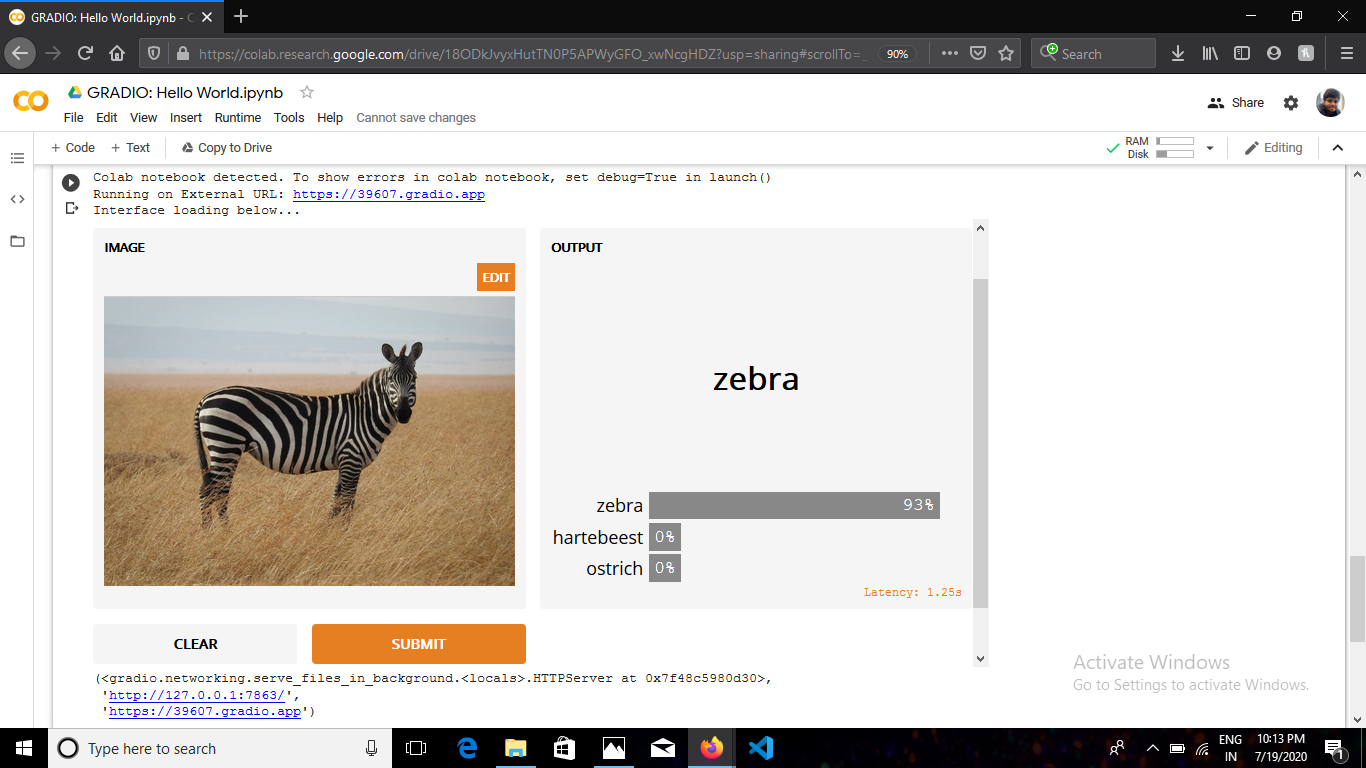Also if you copy the link and paste it in your browser, your interface will look like this: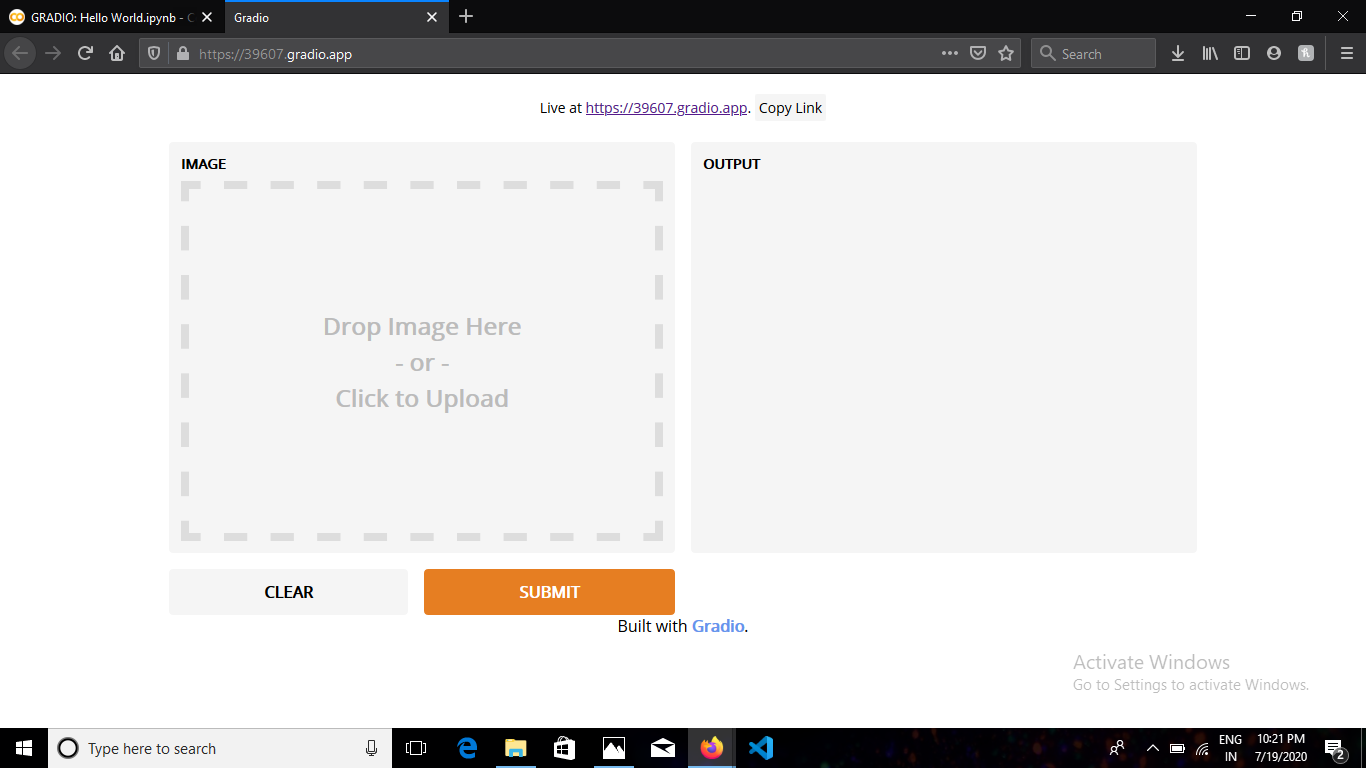It is not localhost so you can open the same link on any device.

Attention geek! Strengthen your foundations with the Python Programming Foundation Course and learn the basics.

To begin with, your interview preparations Enhance your Data Structures concepts with the Python DS Course.

My Personal Notes arrow_drop_upCheck out this Author's contributed articles.

If you like GeeksforGeeks and would like to contribute, you can also write an article using contribute.geeksforgeeks.org or mail your article to contribute@geeksforgeeks.org. See your article appearing on the GeeksforGeeks main page and help other Geeks.

Please Improve this article if you find anything incorrect by clicking on the "Improve Article" button below.